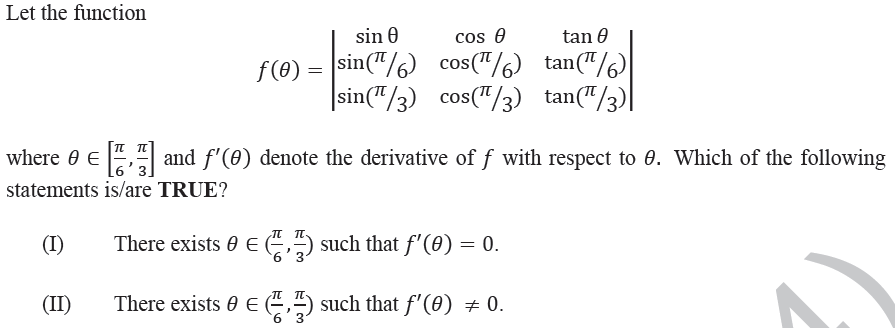Open In App

# GATE | GATE-CS-2014-(Set-1) | Question 16(A) I only
(B) II only
(C) Both I and II
(D) Neither I nor II

Explanation: In the given question,ranges fromto.
When=, the value of the function is 0, since Rows 1 and 2 are the same.
When=, the value of the function is 0, since Rows 1 and 3 are the same.
Therefore,There exists a valueof such that. So the first statement is true.
Also there may also exist a value, such that. This is because the function is not a constant function, i.e. all values in the range are not equal to.